# Introduction to the evclust package

#### 2016-08-28

The package evclust contains methods for evidential clustering. In evidential clustering, cluster membership uncertainty is represented by Dempster-Shafer mass functions. The user is invited to read the papers cited in this vignette to get familiar with the main concepts underlying evidential clustering. These papers can be downloaded from the author’s web site, at https://www.hds.utc.fr/~tdenoeux. Here, we provide a guided tour of the main functions in the evclust package. It consists in five main functions, implementing five different evidential clustering algorithms:

• ecm: Evidential c-means algorithm (Masson and Denoeux 2008)
• recm: Relational Evidential c-means algorithm (Masson and Denœux 2009)
• kevclus: $$k$$-EVCLUS algorithm (Denœux and Masson 2004; Denœux, Sriboonchitta, and Kanjanatarakul 2016)
• EkNNclus: E$$k$$-NNclus algorithm (Denœux, Kanjanatarakul, and Sriboonchitta 2015)
• cecm: Constrained Evidential c-means algorithm (Antoine et al. 2012)

You first need to install this package:

library(evclust)

## Evidential c-means (ECM) algorithm

The Evidential $$c$$-means (ECM) algorithm (Masson and Denoeux 2008) is a $$c$$-means-like algorithm that minimizes a cost function by searching alternatively the space of prototypes and the space of credal partitions. Unlike the hard and fuzzy $$c$$-means algorithms, ECM associates a prototype not only to clusters, but also to sets of clusters. The prototype associated to a set of clusters is defined as the barycenter of the prototypes of each single cluster in the set. The cost function to be minimized insures that objects close to a prototype have a large mass assigned to the corresponding set of clusters.

Consider, for instance, the fourclass data. This dataset consists in four clusters in a two-dimensional space.

data(fourclass)
x<-fourclass[,1:2]
y<-fourclass[,3]
plot(x[,1],x[,2],pch=y,col=y,xlab=expression(x),ylab=expression(x))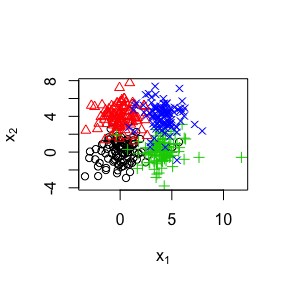We can run ECM with c=4 clusters on this data as follows:

clus<-ecm(x,c=4,type='full',alpha=1,beta=2,delta=sqrt(20),disp=FALSE)

The option type='full' is actually the option. It implies that mass functions in the credal partition will have $$2^c$$ focal sets. You can get basic information about the credal partition using the method summary:

summary(clus)
## ------ Credal partition ------
## 4 classes,400 objects
## Generated by ecm
## Focal sets:
##       [,1] [,2] [,3] [,4]
##  [1,]    0    0    0    0
##  [2,]    1    0    0    0
##  [3,]    0    1    0    0
##  [4,]    1    1    0    0
##  [5,]    0    0    1    0
##  [6,]    1    0    1    0
##  [7,]    0    1    1    0
##  [8,]    1    1    1    0
##  [9,]    0    0    0    1
## [10,]    1    0    0    1
## [11,]    0    1    0    1
## [12,]    1    1    0    1
## [13,]    0    0    1    1
## [14,]    1    0    1    1
## [15,]    0    1    1    1
## [16,]    1    1    1    1
## Value of the criterion=265.38
## Nonspecificity=0.32
## Prototypes:
##            [,1]       [,2]
## [1,] -0.5347741  4.1815659
## [2,] -0.4527994 -1.0651201
## [3,]  4.4345007  4.6390425
## [4,]  4.3301430 -0.2223622
## Number of outliers=1.00

We can restrict the focals set to pairs by changing the type argument.

clus<-ecm(x,c=4,type='pairs',alpha=1,beta=2,delta=sqrt(20),disp=FALSE)
summary(clus)
## ------ Credal partition ------
## 4 classes,400 objects
## Generated by ecm
## Focal sets:
##       [,1] [,2] [,3] [,4]
##  [1,]    0    0    0    0
##  [2,]    1    0    0    0
##  [3,]    0    1    0    0
##  [4,]    0    0    1    0
##  [5,]    0    0    0    1
##  [6,]    1    1    0    0
##  [7,]    1    0    1    0
##  [8,]    1    0    0    1
##  [9,]    0    1    1    0
## [10,]    0    1    0    1
## [11,]    0    0    1    1
## [12,]    1    1    1    1
## Value of the criterion=308.06
## Nonspecificity=0.25
## Prototypes:
##            [,1]        [,2]
## [1,] -0.4748690  4.06096872
## [2,] -0.3475538 -0.89795356
## [3,]  4.3162387  4.56157113
## [4,]  4.1993692 -0.07747143
## Number of outliers=1.00

A plot of the credal partition can be generated as follows:

plot(clus,x,mfrow=c(2,2),ytrue=y,Approx=2)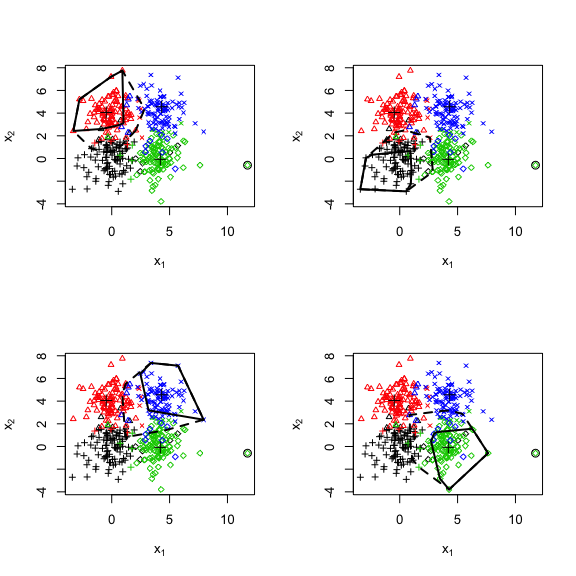In this plot, the lower and upper approximations of each cluster are plotted as solid and interrupted lines, respectively. Since we selected Approx=2, the lower and upper approximations are defined as follows. Let $$A_i$$ be the focal set of mass function $$m_i$$ with the largest mass. The lower approximation of cluster $$\omega_k$$ is the set of objects such that $$A_i=\{\omega_k\}$$, while the upper approximation is the set of objects such that $$\omega_k \in A_i$$. The outliers are the objects such that $$A_i=\emptyset$$. They are displayed as circles in the figure above.

## RECM

The ECM algorithm can only be used with attribute data. The Relational Evidential c-means algorithm (RECM) (Masson and Denœux 2009) is a version of ECM that can handle dissimilarity data, i.e., data that consist in a matrix on dissimilarities between $$n$$ objects. Consider, for instance, the Proteins dataset. It consists of a dissimilarity matrix derived from the structural comparison of 213 protein sequences. Each of these proteins is known to belong to one of four classes of globins: hemoglobin-$$\alpha$$ (HA), hemoglobin-$$\beta$$ (HB), myoglobin (M) and heterogeneous globins (G). We can display these data using Multidimensional Scaling:

data(protein)
z<- cmdscale(protein$D,k=2) plot(z[,1],z[,2],xlab='axis 1',ylab='axis 2')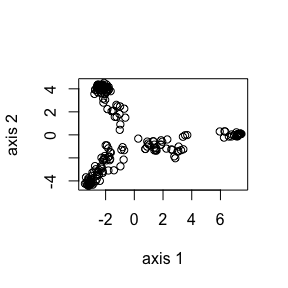We can see that the data points are grouped in four clusters. We can run RECM on this dataset at follows, clus <- recm(D=protein$D,c=4,type='pairs',alpha=0.2,beta=1.1,delta2=20,disp=FALSE)
summary(clus)
## ------ Credal partition ------
## 4 classes,213 objects
## Generated by recm
## Focal sets:
##       [,1] [,2] [,3] [,4]
##  [1,]    0    0    0    0
##  [2,]    1    0    0    0
##  [3,]    0    1    0    0
##  [4,]    0    0    1    0
##  [5,]    0    0    0    1
##  [6,]    1    1    0    0
##  [7,]    1    0    1    0
##  [8,]    1    0    0    1
##  [9,]    0    1    1    0
## [10,]    0    1    0    1
## [11,]    0    0    1    1
## [12,]    1    1    1    1
## Value of the criterion=771.06
## Nonspecificity=0.07
## Number of outliers=0.00
plot(clus,X=z,mfrow=c(2,2),ytrue=protein$y)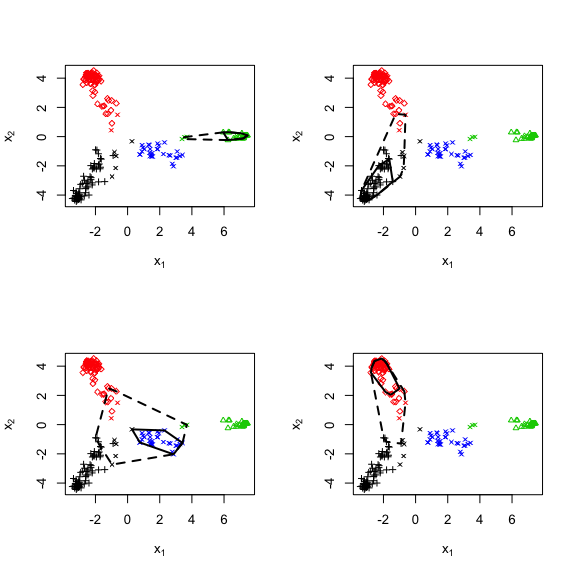## k-EVCLUS The RECM algorithm requires to store the whole dissimilarity matrix. Consequently, it is not suitable to handle large dissimilarity datasets. The k-EVCLUS algorithm, recently introduced in (Denœux, Sriboonchitta, and Kanjanatarakul 2016), overcomes this limitation. It is an improved version of the EVCLUS algorithm introduced in (Denœux and Masson 2004). The EVCLUS algorithm applies ideas from Multidimensional Scaling to clustering: given a dissimilarity matrix, it finds a credal partition such that the degrees of conflict between mass functions match the dissimilarities, dissimilar objects being represented by highly conflicting mass functions; this is achieved by iteratively minimizing a stress function. The k-EVCLUS algorithm uses a more efficient optimization procedure, and uses only the dissimilarities between each object and $$k$$ randomly chosen other objects. As a result, the complexity is reduced from quadratic to linear. As an example, let us generate a dataset from the same distribution as the fourclass dataset, but with $$n=1000$$ objects. n<-1000 x<-matrix(rt(2*n,df=5),n,2) y<-c(rep(1,n/4),rep(2,n/4),rep(3,n/4),rep(4,n/4)) x[(y==2)|(y==4),2]<- 5 + x[(y==2)|(y==4),2] x[(y==3)|(y==4),1]<- 5 + x[(y==3)|(y==4),1] plot(x[,1],x[,2],pch=y,col=y,xlab=expression(x),ylab=expression(x))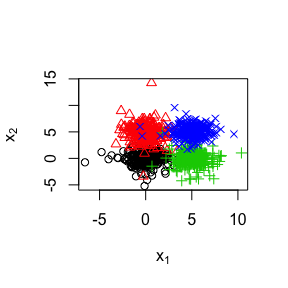There are $$1000\times 999/2=499,500$$ pairwises distances for these data. However, by choosing $$k=50$$, we can use only $$1000\times 50=50,000$$ distances, i.e., only 10 percent of the original distances to generate a credal partition. clus<-kevclus(x=x,c=4,k=50,disp=FALSE) ##  1.00000000 0.01841452 0.01841452 summary(clus) ## ------ Credal partition ------ ## 4 classes,1000 objects ## Generated by kevclus ## Focal sets: ## [,1] [,2] [,3] [,4] ## [1,] 0 0 0 0 ## [2,] 1 0 0 0 ## [3,] 0 1 0 0 ## [4,] 0 0 1 0 ## [5,] 0 0 0 1 ## [6,] 1 1 1 1 ## Value of the criterion=0.02 ## Nonspecificity=0.13 ## Number of outliers=11.00 For a partition created by kevclus, the plot.credpart functions generates two plots: the usual plot of the credal partition in the attribute space, and the Shepard diagram. This is a plot of the degrees of conflict vs. the transformed dissimilarities. If the argument X is not supplied, only the Shepard diagram is plotted. plot(clus,X=x,ytrue=y)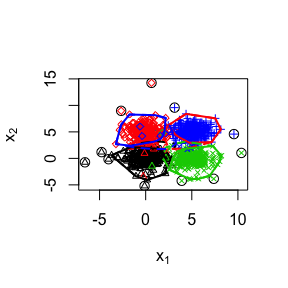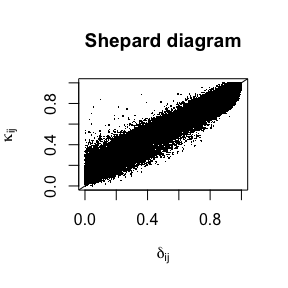## EKNN-clus The clustering methods described above require the number of cluters to be fixed in advance. In contrast, EK-NNclus (Denœux, Kanjanatarakul, and Sriboonchitta 2015) is another evidential clustering algorithm that automatically determines the number of clusters. Starting from an initial partition, E$$K$$-NNclus iteratively reassigns objects to clusters using the E$$K$$-NN rule, until a stable partition is obtained. After convergence, the cluster membership of each object is described by a Dempster-Shafer mass function assigning a mass to each cluster and to the whole set of clusters. The mass assigned to the set of clusters can be used to identify outliers. The method can be implemented in a competitive Hopfield neural network, whose energy function is related to the plausibility of the partition. The procedure can thus be seen as searching for the most plausible partition of the data. The E$$K$$-NNclus algorithm depends on two parameters, the number $$K$$ of neighbors and a scale parameter $$q$$, which can be fixed using simple heuristics. As an example, let us apply E$$K$$-NNclus to the s2 dataset. This dataset contains 5000 two-dimensional vectors grouped in 15 Gaussian clusters. data(s2) plot(s2[,1],s2[,2],xlab=expression(x),ylab=expression(x),pch='.')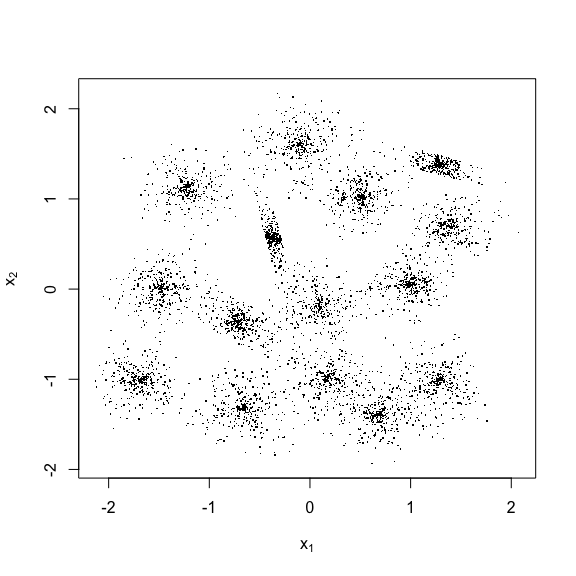To apply E$$K$$-NNclus, we need to fix $$k$$ and $$q$$. Results presented (Denœux, Kanjanatarakul, and Sriboonchitta 2015) suggest that a value of $$K$$ of the order of two or there times $$\sqrt{n}$$ and $$q$$ greater than 0.5 are suitable. For instance, let use set $$K=200$$ and $$q=0.9$$. The algorithm is initialized with a random partition in 500 clusters. It is run ntrials=5 times, and the result with the lowest value of the energy function is kept. y0<-sample(500,size=5000,replace=TRUE) clus<- EkNNclus(s2,K=200,y0=y0,ntrials=5,q=0.9,disp=FALSE) ## Summary of the partition summary(clus) ## ------ Credal partition ------ ## 15 classes,5000 objects ## Generated by EkNNclus ## Focal sets: ## [,1] [,2] [,3] [,4] [,5] [,6] [,7] [,8] [,9] [,10] [,11] [,12] [,13] ## [1,] 0 0 0 0 0 0 0 0 0 0 0 0 0 ## [2,] 1 0 0 0 0 0 0 0 0 0 0 0 0 ## [3,] 0 1 0 0 0 0 0 0 0 0 0 0 0 ## [4,] 0 0 1 0 0 0 0 0 0 0 0 0 0 ## [5,] 0 0 0 1 0 0 0 0 0 0 0 0 0 ## [6,] 0 0 0 0 1 0 0 0 0 0 0 0 0 ## [7,] 0 0 0 0 0 1 0 0 0 0 0 0 0 ## [8,] 0 0 0 0 0 0 1 0 0 0 0 0 0 ## [9,] 0 0 0 0 0 0 0 1 0 0 0 0 0 ## [10,] 0 0 0 0 0 0 0 0 1 0 0 0 0 ## [11,] 0 0 0 0 0 0 0 0 0 1 0 0 0 ## [12,] 0 0 0 0 0 0 0 0 0 0 1 0 0 ## [13,] 0 0 0 0 0 0 0 0 0 0 0 1 0 ## [14,] 0 0 0 0 0 0 0 0 0 0 0 0 1 ## [15,] 0 0 0 0 0 0 0 0 0 0 0 0 0 ## [16,] 0 0 0 0 0 0 0 0 0 0 0 0 0 ## [17,] 1 1 1 1 1 1 1 1 1 1 1 1 1 ## [,14] [,15] ## [1,] 0 0 ## [2,] 0 0 ## [3,] 0 0 ## [4,] 0 0 ## [5,] 0 0 ## [6,] 0 0 ## [7,] 0 0 ## [8,] 0 0 ## [9,] 0 0 ## [10,] 0 0 ## [11,] 0 0 ## [12,] 0 0 ## [13,] 0 0 ## [14,] 0 0 ## [15,] 1 0 ## [16,] 0 1 ## [17,] 1 1 ## Value of the criterion=547801.98 ## Nonspecificity=0.00 ## Number of outliers=0.00 ## Plot of the partition plot(clus,X=s2,Outliers=FALSE)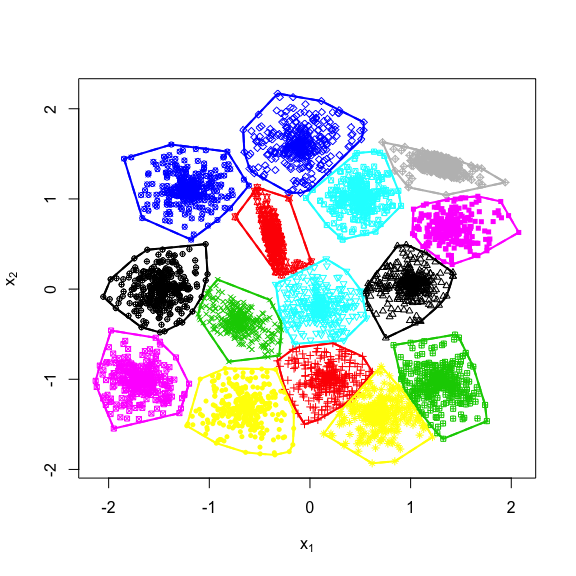We can see that E$$K$$-NNclus generally finds 15 or 14 clusters for these data. In constrast with the previous algorithms, E$$K$$-NNclus generates a credal partition with normalized mass functions. Outliers tend to have a large mass $$m(\Omega)$$ on the frame of discernment, but the credal partition is otherwise usually very close to a hard partition. ## Generating a credal partition with a large number of clusters If no restriction is imposed on the focal sets, the number of parameters to be estimated in evidential clustering grows exponentially with the number $$c$$ of clusters, which makes it intractable unless $$c$$ is small. If we allow masses to be assigned to pairs of clusters, as suggested in [@denoeux04b] and [@masson08], the number of focal sets becomes proportional to $$c^2$$, which is manageable for moderate values of $$c$$ (say, until 10), but still impractical for larger $$n$$. It is clear, however, that only a few pairs of contiguous clusters will be assigned some mass during the learning process. To determine which pairs of clusters can potentially become focal sets, we can use the following three-step approach (Denœux, Sriboonchitta, and Kanjanatarakul 2016): 1. In the first step, a clustering algorithm (ecm, recm or kevclus) is run in the basic configuration, with focal sets of cardinalities 0, 1 and (optionally) $$c$$. A credal partition $$M_0$$ is obtained. 2. The similarity between each pair of clusters $$(\omega_j,\omega_\ell)$$ is analyzed, and we determine the set $$P_K$$ of pairs $$\{\omega_j,\omega_\ell\}$$ that are mutual $$K$$ nearest neighbors. 3. The clustering algorithm is run again, starting from the previous credal partition $$M_0$$, and adding as focal sets the pairs in $$P_K$$. As an example, consider again the s2 dataset with the ecm algorithm. We first construct a credal partition with $$c=15$$ clusters and $$f=16$$ focal sets (the empty set and the sigletons): data(s2) clus<-ecm(x=s2,c=15,type='simple',Omega=FALSE,delta=1,disp=FALSE) plot(x=clus,X=s2,Outliers = TRUE)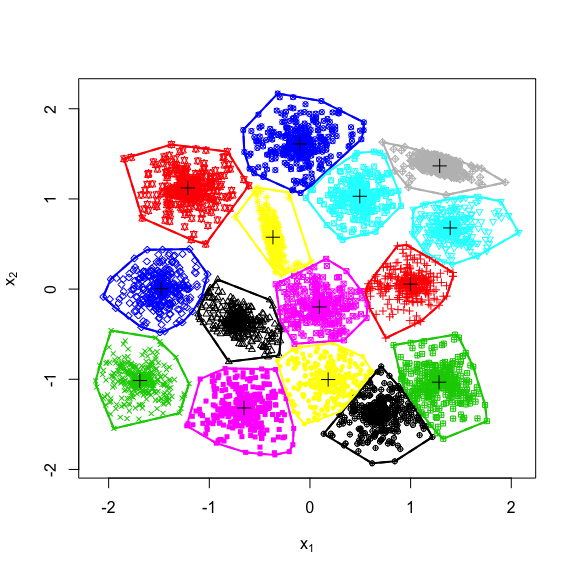We then search for pairs of clusters that are $$K=2$$ mutual nearest neighbors: P<-createPairs(clus,k=2) P$pairs
##       row col
##  [1,]   1   4
##  [2,]   3   4
##  [3,]   2   5
##  [4,]   1   6
##  [5,]   5   8
##  [6,]   7  10
##  [7,]   9  11
##  [8,]   8  13
##  [9,]  12  13
## [10,]   9  15
## [11,]  14  15

Finally, we run again ecm, with the pairs of clusters found in the previous step:

clus1<-ecm(x=s2,c=15,type='pairs',Omega=FALSE,pairs=P$pairs,g0=clus$g,delta=1,disp=FALSE)
plot(x=clus1,X=s2,Outliers = TRUE,Approx=2)